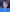# Physics - Introduction to the Fundamentals

February 9, 2021

**Disclaimer: this is not a coherent lesson plan in any way, I'm just tranferring notes from my physical notebook to here for my personal retention. This is not really meant for anyone's learning purpose because the diagrams I drew with my side comments are not being transferred here. Hope you enjoy anyway! :D

# What is physics?

It’s difficult to define exactly what physics is. For one, physics keeps changing as we progress and make new discoveries. New theories don't just bring new answers. They also create new questions that might not have even made sense when viewed from within the previous theory of physics. Although this is what makes physics so cool, it also forces attempts at defining physics into generalizations about what physics has been rather than what it might be at some point in the future.

So, I'll break down what physicists actually try to do:

I. Precisely define the most fundamental measurable quantities in the universe (e.g., velocity, electric field, kinetic energy). The effort to find the most fundamental description of the universe is a quest that has historically always been a big part of physics.

II. Find relationships between those fundamental measured quantities (e.g., Newton’s Laws, conservation of energy, special relativity). These patterns and correlations are expressed using words, equations, graphs, charts, diagrams, models, and any other means that allow us to express a relationship in a way that we as humans can better understand and use. Math makes things simpler.

Trying to describe an extremely complex universe with clarifying laws is what physics is all about :)

# Let's define some key terms

Coordinate system - System we define to describe position. The origin is the point we label as zero. Coordinate systems also define directions for positive and negative numbers.

Position - Location of an object relative to the origin. We often use the symbol "x" to refer to position.

Displacement - The change in position of an object. We use the symbol Δx for displacement, where Δ means "change." A vector quantity with units of distance.

Distance - Total amount the object has moved. This depends on the whole path traveled, not just the starting and ending points. Distance traveled is always a non-negative number. A scalar quantity with units of distance.

Reference frame / frame of reference - A point of view from which measurements can be made. All frames of reference are equally valid.

Scalar Quantity - A distance that only shows magnitude, not direction

Vector Quantity - A distance that shows magnitude and direction

# Rules to Know

## Distance, Displacement, Velocity

• Distance is always positive, displacement can be negative and positive
• Distance is the amount total traveled
• Displacement is the exact amount of units it took to get to the final position
• Scalar quantities are the speed and distance, where they will always be positive
• Vector quantities are the velocity and displacement where they can also be negative, depending on the direction
• D = VT where D = distance or displacement AND V = velocity or speed. T = time
• Average speed = distance / time where Average velocity = displacement / time

## Acceleration

• Acceleration tells you how fast the speed -- or really, the velocity is changing
• acceleration - change in velocity / change in time
• final velocity - initial velocity / time = change in velocity
• Any time the acceleration is positive, velocity will increase. Negative acceleration makes negative velocity
• Vf = V0 + at which means that the final velocity = initial velocity + acceleration(time)

## Gravitational Acceleration and Projectile Motion

• G = -9.8 m/2^2 is the gravitational acceleration for the earth, it's a solid rule
• Projectile Motion - a projectile is an object that is moving under the influence of gravity (friction and air resistance is ignored)
• Gravitational acceleration affects vertical acceleration, not horizontal acceleration

## Newton's Laws of Motion

• 1st law of motion - An object at rest will remain at rest unless acted on by a force. An object in motion will continue in motion unless acted on by a net force.

• 2nd law of motion - The net force of an object is equal to the mass times the acceleration.

• F = m*a /// Fnet = mass * acceleration
• 3rd law of motion - for every action (force) in nature there is an equal and opposite reaction. In other words, if object A exerts a force on object B, then object B also exerts an equal and opposite force on object AHey, I'm Snigdha! I like to understand things and explain them well to others — this is my commitment to self-assigned learning. Say hello on twitter! 👋